# COULOMB'S LAW - Definition with example.

COULOMB'S LAW

The electrostatic force of interaction between two static point electric charges is directly proportional to the product of the charges, inversely proportional to the square of the distance between them and acts along the straight line joining the two charges.

If two points charges q1 and q2 separated by a distance r. Let F be the electrostatic force between these two charges. According to Coulomb's law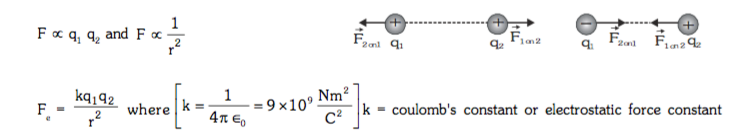Coulomb's law in vector form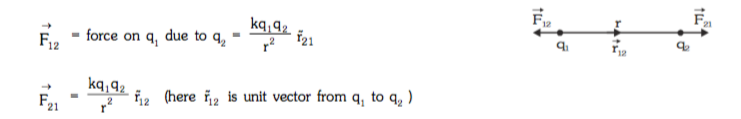Coulomb's law in terms of position vector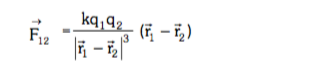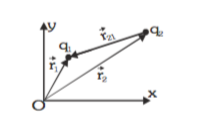Principle of superposition

The force is a two body interaction, i.e., electrical force between two point charges is independent of presence

or absence of other charges and so the principle of superposition is valid, i.e., force on a charged particle due

to number of point charges is the resultant of forces due to individual point charges, i.e., F1 = F12 + F13 +... Note : Nuclear force is many body interaction, so principle of superposition is not valid in case of nuclear force.

When a number of charges are interacting, the total force on a given charge is vector sum of the forces exerted on it by all other charges individually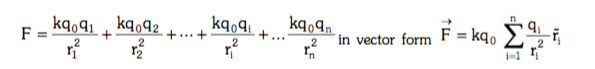Example :

If the distance between two equal point charges is doubled and their individual charges are also doubled, what would happen to the force between them?

Solution :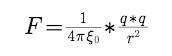Again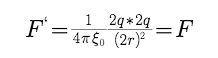So, the force will remain the same.

Example :

A particle of mass m carrying charge '+q1' is revolving around a fixed charge '–q2' in a circular path of radius r. Calculate the period of revolution.

Solution :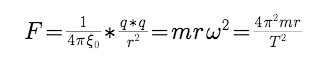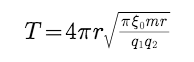where r is the vector drawn from source charge is test charge.

Post By : Preeti Rai 18 Apr, 2019 3420 views Physics